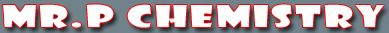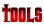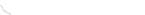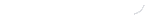activity seriesbond energybond lengthscalculatorcommon namesconversion factors: energyconversion factors: lengthconversion factors: massconversion factors: pressureconversion factors: temp.conversion factors: volumecovalent prefixesdensity solverdiatomic elementse-config. chartelement listgas law formulasKa's of polyprotic acidsKa's of weak acidsKb's of weak basesmetric conversion chartmole conversion chartmolecular geometriesorganic prefixesperiodic tableperiodic table (flash)pH/pOH converterpolyatomic ionspressure converterSI unitssolubility chartsolubility of salts rulessolubility product constantsstoichiometry charttemp. conversiontemp. formulasthermodynamic datavapor pressure of water
All Tutorials

 Atomic Number Atomic Radius Trend Atomic Weight Balancing Chemical Equations Binary Ionic Compounds Boiling Point Trend Calculating Entropy Calories and Joules Chemical Equations Complete Combustion Compounds in Crust of Earth Decomposition of a Carbonate Decomposition of a Chlorate Decomposition of a Hydrate Decomposition Reactions Density Determining the Formula of a Hydrate Dilutions Double Replacement Reactions Electron Configuration of Ions Electron Configuration Principles Electron Configuration Shortcut Electronegativity Trend Elements in Crust of Earth Empirical Formula Gak Recipe Gram Formula Mass Heat of Reaction History of the Atom History of the Periodic Table How to Name Covalent Compounds How To Round Numbers How to Write Covalent Compound Formulas Ionic Compounds Using the Common Naming System Ionic Compounds Using the Stock Naming System Ionic Compounds with Polyatomic Ions Ionization Energy Trend Isotopes Lewis Dot Diagrams Mass Composition Mass Number Melting Point Trend Metric Conversion Molality Molarity Mole Fraction Molecular Formula Naming Hydrates Naming Ionic Compounds with Multivalent Cations Old Element Names Oxidation Numbers of Elements in a Covalent Compound Percent Composition Percent Water in a Hydrate Period Table Organization Properties of Salts Proton Neutron and Electron Quick Facts Salts and Metals Scientific Notation SI Units Significant Figure Calculations Significant Figures Single Replacement Reactions Slime Recipe Spontaneous Reactions Synthesis Reactions